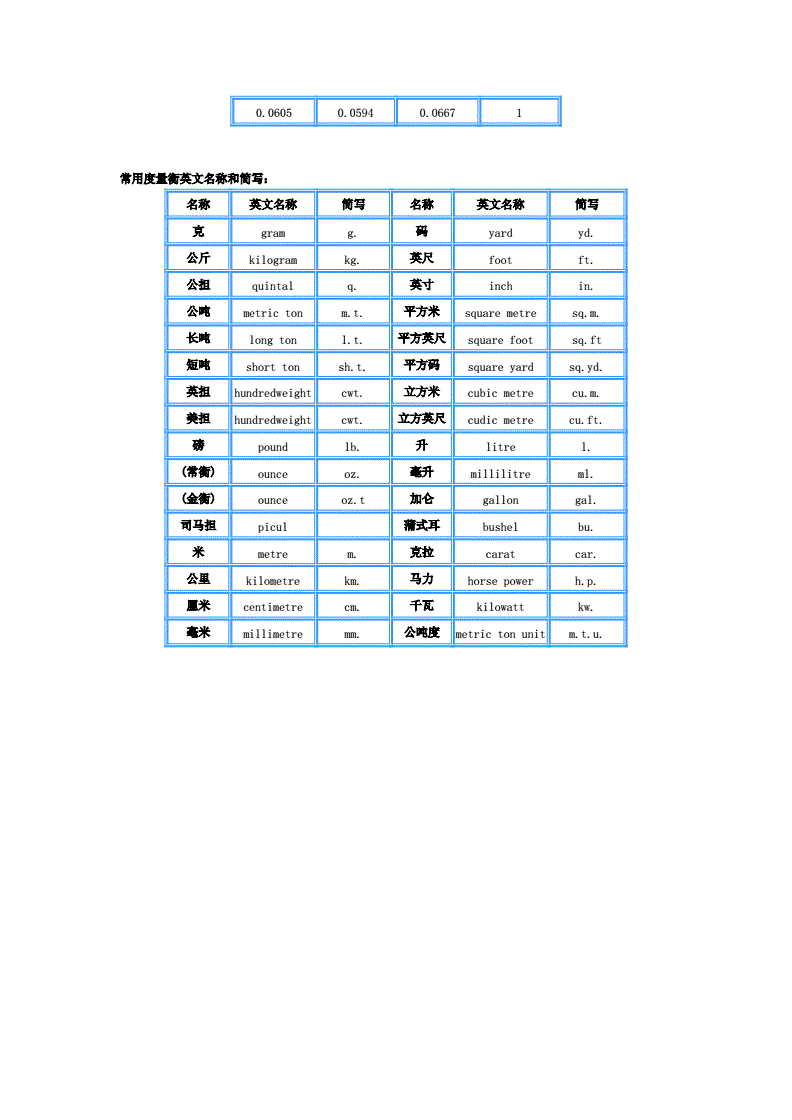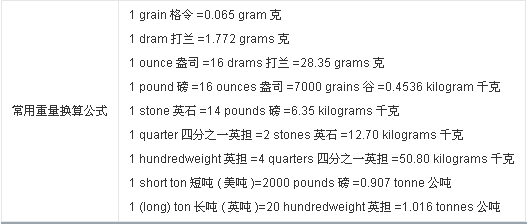# 1磅多少斤(155磅多少斤)1米=3.281英尺

1英寸=25.4毫米

1磅=0.4536公斤

1公斤力=9.81牛顿

1磅力=4.45牛顿

1兆帕=145.161磅/英寸

W= 0.006165×d×d

d = 直径mm

W= 0.00617 ×d×d

d= 断面直径mm

W= 0.00785×a×a

a= 边宽mm

W= 0.00785×b×d

b= 边宽mm

d= 厚mm

W= 0.006798×s×s

s= 对边距离mm

W= 0.0065×s×s

s= 对边距离mm

W= 0.00785×[d（2b–d）+0.215（R2– 2r2）]

b= 边宽

d= 边厚

R= 内弧半径

r= 端弧半径

W=0.00785×[d（B+b – d）+0.215（R2–2r 2）]

B= 长边宽

b= 短边宽

d= 边厚R= 内弧半径

r= 端弧半径

W=0.00785×[hd+2t （b–d）+0.349（R2–r2）]

h= 高

b= 腿长

d= 腰厚

t= 平均腿厚

R= 内弧半径

r= 端弧半径

W=0.00785×[hd+2t （b–d）+0.615（R2–r 2）]

h= 高

b= 腿长

d= 腰厚

t= 平均腿厚

R= 内弧半径

r= 端弧半径

W= 7.85×d

d= 厚

W= 0.02466×S（D– S）

D= 外径

S= 壁厚

1.铁线：直径mm*直径mm*0.00617*长度mm/1000=重量（kg）

2.铁板：厚度mm*宽度mm*长度mm*0.00785/1000=重量 (kg)

3.圆管：(外径mm-厚度mm)*厚度mm*长度mm*0.02466/1000=重量 (kg)

4.长方管：[(长边+短边)*2/3.1416-厚度]mm*厚度mm*长度mm*0.02466/1000=重量（kg）

5.正方管：（边长*4/3.1416-厚度）mm*厚度mm*长度mm*0.02466/1000=重量 (kg)

【其他有色金属】

1）钢管重量(kg)=0.00617*直径*直径*长度；

2）方钢重量(kg)=0.00785*边宽*边宽*长度；

3）六角钢重量(kg)=0.0068*对边宽*对边宽*长度；

4）八角钢重量(kg)=0.0065*对边宽*对边宽*长度；

5）螺纹钢重量(kg)=0.00617*计算直径*计算直径*长度

6）角钢重量(kg)=0.00785*(边宽+边宽-边厚)*边厚*长度；

7）扁钢重量(kg)=0.0075*厚度*边宽*长度；

8）钢管重量(kg)=0.02466*壁厚*(外径-壁厚)*长度；

9）钢板重量(kg)=7.85*厚度*面积；

10）圆紫铜棒重量(kg)=0.00698*直径*直径*长度；

11)圆黄铜棒重量(kg)=0.00668*直径*直径*长度；

12）圆铝棒重量(kg)=0.0022*直径*直径*长度；

13）方紫铜棒重量(kg)=0.0089*边宽*边宽*长度；

14）方黄铜棒重量(kg)=0.0085*边宽*边宽*边度；

15）方铝棒重量(kg)=0.0028*边宽*边宽*长度；

16）六角紫铜棒重量(kg)=0.0077*对边宽*对边宽*长度；

17）六角黄铜棒重量(kg)=0.00736*边宽*对边宽*长度；

18）六角铝棒重量(kg)=0.00242*对边宽*对边宽*长度；

19）紫铜板重量(kg)=0.0089*厚*宽*长度；

20）黄铜板重量(kg)=0.0085*厚*宽*长度；

21）铝板重量(kg)=0.00171*厚*宽*长度；

22）圆紫铜管重量(kg)=0.028*壁厚*(外径-壁厚)*长度；

23）圆黄铜管重量(kg)=0.0267*壁厚*(外径-壁厚)*长度；

24）圆钢重量(kg)=0.00617*直径*直径*长度；

25）圆铝管重量(kg)=0.00879*壁厚*(外径-壁厚)*长度；

26）无缝管重量(kg)=0.02466*壁厚*(外径-壁厚)*长度；

27）不锈钢管重量(kg/m)=0.02491*壁厚*(外径-壁厚)

28）角钢每米重量=0.00785*(边宽+边宽-边厚)*边厚

（备注：公式中长度单位为米，面积单位为平方米，其余单位均为毫米）

■■■